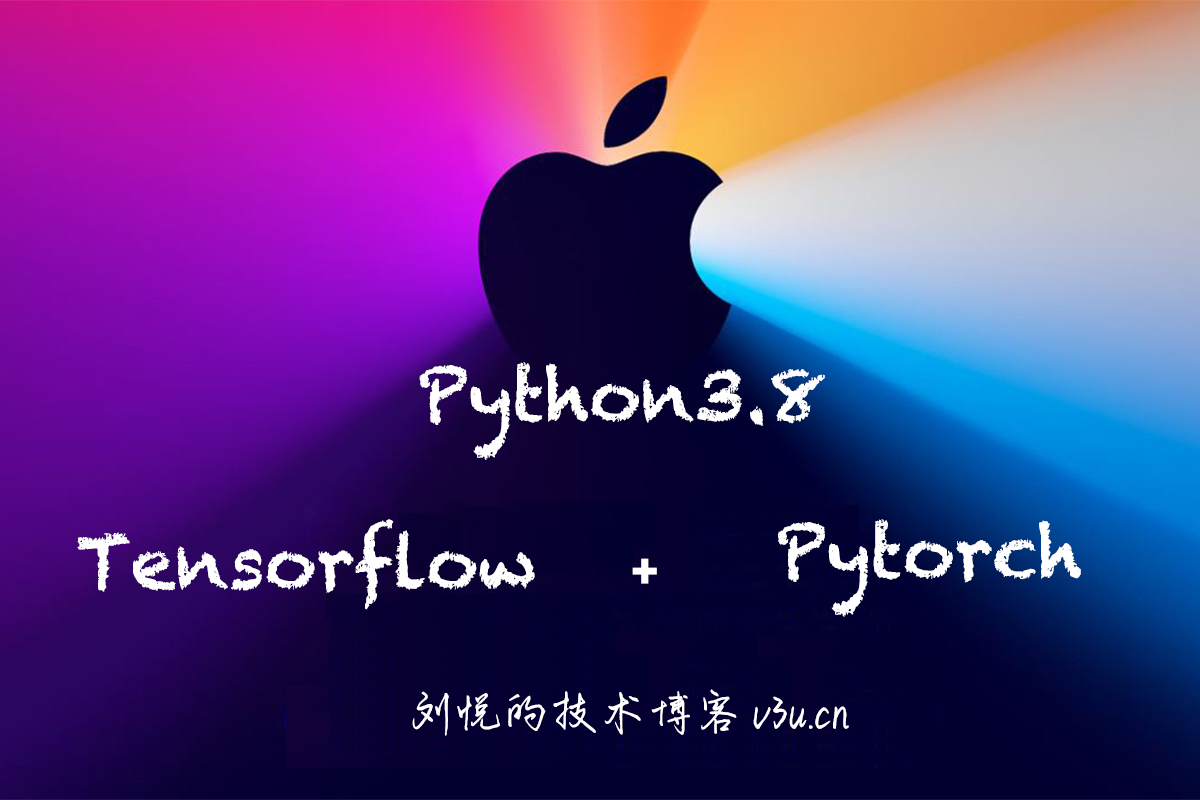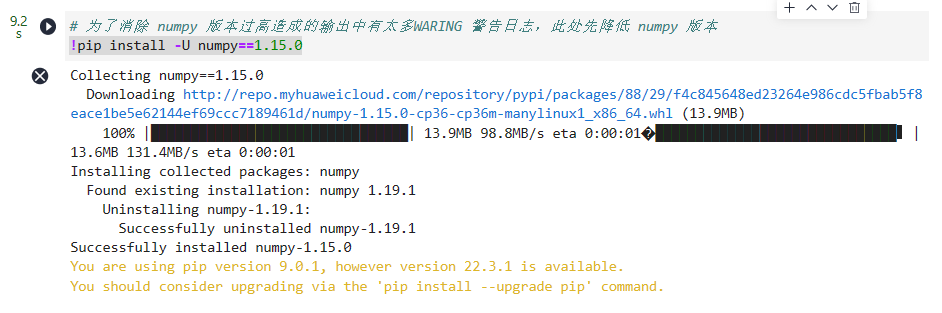# TensorFlow 学习指南 一、基础

+关注继续查看

## 变量

TensorFlow 是一种表示计算的方式，直到请求时才实际执行。 从这个意义上讲，它是一种延迟计算形式，它能够极大改善代码的运行：

• 更快地计算复杂变量
• 跨多个系统的分布式计算，包括 GPU。
• 减少了某些计算中的冗余

x = 35
y = x + 5
print(y)


python basic_script.py


python Code/basic_script.py


(tensorenv)username@computername:~$ 如果起作用，让我们将其转换为 TensorFlow 等价形式。 import tensorflow as tf x = tf.constant(35, name='x') y = tf.Variable(x + 5, name='y') print(y)  运行之后，你会得到一个非常有趣的输出，类似于<tensorflow.python.ops.variables.Variable object at 0x7f074bfd9ef0>。 这显然不是40的值。 原因在于，我们的程序实际上与前一个程序完全不同。 这里的代码执行以下操作： • 导入tensorflow模块并将其命名为tf • 创建一个名为x的常量值，并为其赋值35 • 创建一个名为y的变量，并将其定义为等式x + 5 • 打印y的等式对象 微妙的区别是，y没有像我们之前的程序那样，给出x + 5的当前值”。 相反，它实际上是一个等式，意思是“当计算这个变量时，取x的值（就像那样）并将它加上5”。 y值的计算在上述程序中从未实际执行。 我们来解决这个问题： import tensorflow as tf x = tf.constant(35, name='x') y = tf.Variable(x + 5, name='y') model = tf.global_variables_initializer() with tf.Session() as session: session.run(model) print(session.run(y))  我们删除了print(y)语句，而是创建了一个会话，并实际计算了y的值。这里有相当多的样板，但它的工作原理如下： • 导入tensorflow模块并将其命名为tf • 创建一个名为x的常量值，并为其赋值35 • 创建一个名为y的变量，并将其定义为等式x + 5 • 使用tf.global_variables_initializer()初始化变量（我们将在此详细介绍） • 创建用于计算值的会话 • 运行第四步中创建的模型 • 仅运行变量y并打印出其当前值 上面的第四步是一些魔术发生的地方。在此步骤中，将创建变量之间的依赖关系的图。在这种情况下，变量y取决于变量x，并且通过向其添加5来转换它的值。请记住，直到第七步才计算该值，在此之前，仅计算等式和关系。 1）常量也可以是数组。预测此代码将执行的操作，然后运行它来确认： import tensorflow as tf x = tf.constant([35, 40, 45], name='x') y = tf.Variable(x + 5, name='y') model = tf.global_variables_initializer() with tf.Session() as session: session.run(model) print(session.run(y))  生成包含 10,000 个随机数的 NumPy 数组（称为x），并创建一个存储等式的变量。你可以使用以下代码生成 NumPy 数组： import numpy as np data = np.random.randint(1000, size=10000)  然后可以使用data变量代替上面问题 1 中的列表。 作为一般规则，NumPy 应该用于更大的列表/数字数组，因为它具有比列表更高的内存效率和更快的计算速度。 它还提供了大量的函数（例如计算均值），通常不可用于列表。 3）你还可以在循环更新的变量，稍后我们将这些变量用于机器学习。 看看这段代码，预测它会做什么（然后运行它来检查）： import tensorflow as tf x = tf.Variable(0, name='x') model = tf.global_variables_initializer() with tf.Session() as session: session.run(model) for i in range(5): x = x + 1 print(session.run(x))  4）使用上面（2）和（3）中的代码，创建一个程序，计算以下代码行的“滑动”平均值：np.random.randint(1000)。 换句话说，保持循环，并在每个循环中，调用np.random.randint(1000)一次，并将当前平均值存储在在每个循环中不断更新变量中。 5）使用 TensorBoard 可视化其中一些示例的图。 要运行 TensorBoard，请使用以下命令：tensorboard --logdir=path/to/log-directory import tensorflow as tf x = tf.constant(35, name='x') print(x) y = tf.Variable(x + 5, name='y') with tf.Session() as session: merged = tf.summary.merge_all() writer = tf.summary.FileWriter("/tmp/basic", session.graph) model = tf.global_variables_initializer() session.run(model) print(session.run(y))  要了解 Tensorboard 的更多信息，请访问我们的可视化课程 ## 数组 在本教程中，我们将处理图像，以便可视化数组的更改。 数组是强大的结构，我们在前面的教程中简要介绍了它。 生成有趣的数组可能很困难，但图像提供了很好的选择。 首先，下载此图像到你的计算机（右键单击，并寻找选项“图片另存为”）。此图片来自维基共享的用户 Uoaei1 要处理图像，我们需要matplotlib。 我们还需要pillow库，它会覆盖已弃用的 PIL 库来处理图像。 你可以使用 Anaconda 的安装方法在你的环境中安装它们： conda install matplotlib pillow  要加载图像，我们使用matplotlib的图像模块： import matplotlib.image as mpimg import os # 首先加载图像 dir_path = os.path.dirname(os.path.realpath(__file__)) filename = dir_path + "/MarshOrchid.jpg" # 加载图像 image = mpimg.imread(filename) # 打印它的形状 print(image.shape)  上面的代码将图像作为 NumPy 数组读入，并打印出大小。 请注意，文件名必须是下载的图像文件的完整路径（绝对路径或相对路径）。 你会看到输出，即(5528, 3685, 3)。 这意味着图像高 5528 像素，宽 3685 像素，3 种颜色“深”。 你可以使用pyplot查看当前图像，如下所示： import matplotlib.pyplot as plt plt.imshow(image) plt.show()  现在我们有了图像，让我们使用 TensorFlow 对它进行一些更改。 ## 几何操作 我们将要执行的第一个转换是转置，将图像逆时针旋转 90 度。 完整的程序如下，其中大部分是你见过的。 import tensorflow as tf import matplotlib.image as mpimg import matplotlib.pyplot as plt import os # 再次加载图像 dir_path = os.path.dirname(os.path.realpath(__file__)) filename = dir_path + "/MarshOrchid.jpg" image = mpimg.imread(filename) # 创建 TF 变量 x = tf.Variable(image, name='x') model = tf.global_variables_initializer() with tf.Session() as session: x = tf.transpose(x, perm=[1, 0, 2]) session.run(model) result = session.run(x) plt.imshow(result) plt.show()  转置操作的结果：新东西是这一行： x = tf.transpose(x, perm=[1, 0, 2])  该行使用 TensorFlow 的transpose方法，使用perm参数交换轴 0 和 1（轴 2 保持原样）。 我们将要做的下一个操作是（左右）翻转，将像素从一侧交换到另一侧。 TensorFlow 有一个称为reverse_sequence的方法，但签名有点奇怪。 这是文档所说的内容（来自该页面）： tf.reverse_sequence( input, seq_lengths, seq_axis=None, batch_axis=None, name=None, seq_dim=None, batch_dim=None )  反转可变长度切片。 这个操作首先沿着维度batch_axisinput却偏，并且对于每个切片i，沿着维度seq_axis反转第一个seq_lengths [i]元素。 seq_lengths的元素必须满足seq_lengths [i] <= input.dims [seq_dim]，而seq_lengths必须是长度为input.dims [batch_dim]的向量。 然后，输入切片i给出了沿维度batch_axis的输出切片i，其中第一个seq_lengths [i]切片沿着维度seq_axis被反转。 对于这个函数，最好将其视为： • 根据batch_dim迭代数组。 设置batch_dim = 0意味着我们遍历行（从上到下）。 • 对于迭代中的每个项目 • 对第二维切片，用seq_dim表示。 设置seq_dim = 1意味着我们遍历列（从左到右）。 • 迭代中第n项的切片由seq_lengths中的第n项表示 让我们实际看看它： import numpy as np import tensorflow as tf import matplotlib.image as mpimg import matplotlib.pyplot as plt import os # First, load the image again dir_path = os.path.dirname(os.path.realpath(__file__)) filename = dir_path + "/MarshOrchid.jpg" image = mpimg.imread(filename) height, width, depth = image.shape # Create a TensorFlow Variable x = tf.Variable(image, name='x') model = tf.global_variables_initializer() with tf.Session() as session: x = tf.reverse_sequence(x, [width] * height, 1, batch_dim=0) session.run(model) result = session.run(x) print(result.shape) plt.imshow(result) plt.show()  新东西是这一行： x = tf.reverse_sequence(x, np.ones((height,)) * width, 1, batch_dim=0)  它从上到下（沿着它的高度）迭代图像，并从左到右（沿着它的宽度）切片。 从这里开始，它选取大小为width的切片，其中width是图像的宽度。 译者注： 还有两个函数用于实现切片操作。一个是tf.reverse，另一个是张量的下标和切片运算符（和 NumPy 用法一样）。 代码np.ones((height,)) * width创建一个填充值width的 NumPy 数组。 这不是很有效！ 不幸的是，在编写本文时，似乎此函数不允许你仅指定单个值。 “翻转”操作的结果：1）将转置与翻转代码组合来顺时针旋转。 2）目前，翻转代码（使用reverse_sequence）需要预先计算宽度。 查看tf.shape函数的文档，并使用它在会话中计算x变量的宽度。 3）执行“翻转”，从上到下翻转图像。 4）计算“镜像”，复制图像的前半部分，（左右）翻转然后复制到后半部分。 ## 占位符 到目前为止，我们已经使用Variables来管理我们的数据，但是有一个更基本的结构，即占位符。 占位符只是一个变量，我们将在以后向它分配数据。 它允许我们创建我们的操作，并构建我们的计算图，而不需要数据。 在 TensorFlow 术语中，我们随后通过这些占位符，将数据提供给图。 import tensorflow as tf x = tf.placeholder("float", None) y = x * 2 with tf.Session() as session: result = session.run(y, feed_dict={x: [1, 2, 3]}) print(result)  这个例子与我们之前的例子略有不同，让我们分解它。 首先，我们正常导入tensorflow。然后我们创建一个名为xplaceholder，即我们稍后将存储值的内存中的位置。 然后，我们创建一个Tensor，它是x乘以 2 的运算。注意我们还没有为x定义任何初始值。 我们现在定义了一个操作（y），现在可以在会话中运行它。我们创建一个会话对象，然后只运行y变量。请注意，这意味着，如果我们定义了更大的操作图，我们只能运行图的一小部分。这个子图求值实际上是 TensorFlow 的一个卖点，而且许多其他类似的东西都没有。 运行y需要了解x的值。我们在feed_dict参数中定义这些来运行。我们在这里声明x的值是[1,2,3]。我们运行y，给了我们结果[2,4,6] 占位符不需要静态大小。让我们更新我们的程序，让x可以接受任何长度。将x的定义更改为： x = tf.placeholder("float", None)  现在，当我们在feed_dict中定义x的值时，我们可以有任意维度的值。 代码应该仍然有效，并给出相同的答案，但现在它也可以处理feed_dict中的任意维度的值。 占位符也可以有多个维度，允许存储数组。 在下面的示例中，我们创建一个 3 乘 2 的矩阵，并在其中存储一些数字。 然后，我们使用与以前相同的操作，来逐元素加倍数字。 import tensorflow as tf x = tf.placeholder("float", [None, 3]) y = x * 2 with tf.Session() as session: x_data = [[1, 2, 3], [4, 5, 6],] result = session.run(y, feed_dict={x: x_data}) print(result)  占位符的第一个维度是None，这意味着我们可以有任意数量的行。 第二个维度固定为 3，这意味着每行需要有三列数据。 我们可以扩展它来接受任意数量的None维度。 在此示例中，我们加载来自上一课的图像，然后创建一个存储该图像切片的占位符。 切片是图像的 2D 片段，但每个“像素”具有三个分量（红色，绿色，蓝色）。 因此，对于前两个维度，我们需要None，但是对于最后一个维度，需要 3（或None也能用）。 然后，我们使用 TensorFlow 的切片方法从图像中取出一个子片段来操作。 import tensorflow as tf import matplotlib.image as mpimg import matplotlib.pyplot as plt import os # First, load the image again dir_path = os.path.dirname(os.path.realpath(__file__)) filename = dir_path + "/MarshOrchid.jpg" raw_image_data = mpimg.imread(filename) image = tf.placeholder("uint8", [None, None, 3]) slice = tf.slice(image, [1000, 0, 0], [3000, -1, -1]) with tf.Session() as session: result = session.run(slice, feed_dict={image: raw_image_data}) print(result.shape) plt.imshow(result) plt.show()  译者注：使用下标和切片运算符也可以实现切片。 结果是图像的子片段：1）在官方文档中查看 TensorFlow 中的其他数组函数 2）将图像分成四个“角”，然后再将它拼在一起。 3）将图像转换为灰度。 一种方法是只采用一个颜色通道并显示。 另一种方法是将三个通道的平均值作为灰色。 ## 交互式会话 现在我们有了一些例子，让我们更仔细地看看发生了什么。 正如我们之前已经确定的那样，TensorFlow 允许我们创建操作和变量图。这些变量称为张量，表示数据，无论是单个数字，字符串，矩阵还是其他内容。张量通过操作来组合，整个过程以图来建模。 首先，确保激活了tensorenv虚拟环境，一旦激活，请输入conda install jupyter来安装jupter books 然后，运行jupyter notebook以启动 Jupyter Notebook（以前称为 IPython Notebook）的浏览器会话。 （如果你的浏览器没有打开，请打开它并在浏览器的地址栏中输入localhost:8888。） 单击New（新建），然后单击Notebooks（笔记本）下的Python 3（Python 3）。这将启动一个新的浏览器选项卡。通过单击顶部的Untitled（无标题）为该笔记本命名，并为其命名（我使用Interactive TensorFlow）。 如果你以前从未使用过 Jupyter 笔记本（或 IPython 笔记本），请查看此站点来获得简介。 接下来，和以前一样，让我们创建一个基本的 TensorFlow 程序。 一个主要的变化是使用InteractiveSession，它允许我们运行变量，而不需要经常引用会话对象（减少输入！）。 下面的代码块分为不同的单元格。 如果你看到代码中断，则需要先运行上一个单元格。 此外，如果你不自信，请确保在运行之前将给定块中的所有代码键入单元格。 import tensorflow as tf session = tf.InteractiveSession() x = tf.constant(list(range(10)))  在这段代码中，我们创建了一个InteractiveSession，然后定义一个常量值，就像一个占位符，但具有设置的值（不会改变）。 在下一个单元格中，我们可以求解此常量并打印结果。 print(x.eval())  下面我们关闭打开的会话。 session.close()  关闭会话非常重要，并且很容易忘记。 出于这个原因，我们在之前的教程中使用with关键字来处理这个问题。 当with块完成执行时，会话将被关闭（如果发生错误也会发生这种情况 - 会话仍然关闭）。 现在让我们来看更大的例子。 在这个例子中，我们将使用一个非常大的矩阵并对其进行计算，跟踪何时使用内存。 首先，让我们看看我们的 Python 会话当前使用了多少内存： import resource print("{} Kb".format(resource.getrusage(resource.RUSAGE_SELF).ru_maxrss))  在我的系统上，运行上面的代码之后，使用了 78496 千字节。 现在，创建一个新会话，并定义两个矩阵： import numpy as np session = tf.InteractiveSession() X = tf.constant(np.eye(10000)) Y = tf.constant(np.random.randn(10000, 300))  让我们再看一下我们的内存使用情况： print("{} Kb".format(resource.getrusage(resource.RUSAGE_SELF).ru_maxrss))  在我的系统上，内存使用率跃升至 885,220 Kb - 那些矩阵很大！ 现在，让我们使用matmul将这些矩阵相乘： Z = tf.matmul(X, Y)  如果我们现在检查我们的内存使用情况，我们发现没有使用更多的内存 - 没有实际的Z的计算。 只有当我们求解操作时，我们才真正计算。 对于交互式会话，你可以使用Z.eval()，而不是运行session.run(Z)。 请注意，你不能总是依赖.eval()，因为这是使用“默认”会话的快捷方式，不一定是你要使用的会话。 如果你的计算机比较低级（例如，ram 低于 3Gb），那么不要运行此代码 - 相信我！ Z.eval()  你的计算机会考虑很长一段时间，因为现在它才实际执行这些矩阵相乘。 之后检查内存使用情况会发现此计算已经发生，因为它现在使用了接近 3Gb！ print("{} Kb".format(resource.getrusage(resource.RUSAGE_SELF).ru_maxrss))  别忘了关闭你的会话！ session.close()  注意：我建议使用新的 Jupyter Notebook，因为上面的示例代码可能会被意外再次执行，可能导致计算机崩溃！ 1）创建一个整数值的大矩阵（至少 10,000,000）（例如，使用 NumPy 的randint函数）。 创建矩阵后检查内存使用情况。 然后，使用 TensorFlow 的to_float函数将矩阵转换为浮点值。 再次检查内存使用情况，看到内存使用量增加超过两倍。 “加倍”是由创建矩阵的副本引起的，但是“额外增加”的原因是什么？ 执行此实验后，你可以使用此代码显示图像。 from PIL import Image from io import BytesIO # 从字符串读取数据 im = Image.open(BytesIO(result)) im  提示：确保在每一步之后仔细测量内存使用情况，因为只是导入 TensorFlow 就会使用相当多的内存。 2）使用 TensorFlow 的图像函数将上一个教程中的图像（或其他图像）转换为 JPEG 并记录内存使用情况。 ## 可视化 在本课中，我们将介绍如何使用 TensorBoard 创建和可视化图。 我们在第一课变量中简要地浏览了 TensorBoard 那么什么是 TensorBoard 以及我们为什么要使用它呢？ TensorBoard 是一套 Web 应用程序，用于检查和理解你的 TensorFlow 运行和图。 TensorBoard 目前支持五种可视化：标量，图像，音频，直方图和图。 你将在 TensorFlow 中的计算用于训练大型深度神经网络，可能相当复杂且令人困惑，TensorBoard 将使你更容易理解，调试和优化 TensorFlow 程序。 要实际查看 TensorBoard，请单击此处 这就是 TensorBoard 图的样子：## 基本的脚本 下面我们有了构建 TensorBoard 图的基本脚本。 现在，如果你在 python 解释器中运行它，会返回 63。 import tensorflow as tf a = tf.add(1, 2,) b = tf.multiply(a, 3) c = tf.add(4, 5,) d = tf.multiply(c, 6,) e = tf.multiply(4, 5,) f = tf.div(c, 6,) g = tf.add(b, d) h = tf.multiply(g, f) with tf.Session() as sess: print(sess.run(h))  现在我们在代码末尾添加一个SummaryWriter，这将在给定目录中创建一个文件夹，其中包含 TensorBoard 用于构建图的信息。 with tf.Session() as sess: writer = tf.summary.FileWriter("output", sess.graph) print(sess.run(h)) writer.close()  如果你现在运行 TensorBoard，使用tensorboard --logdir=path/to/logs/directory，你会看到在你给定的目录中，你得到一个名为output的文件夹。 如果你在终端中访问 IP 地址，它将带你到 TensorBoard，然后如果你点击图，你将看到你的图。在这一点上，图遍布各处，并且相当难以阅读。 因此，请命名一些部分来其更更加可读。## 添加名称 在下面的代码中，我们只添加了parameter几次。name=[something]。 这个parameter将接受所选区域并在图形上为其命名。 a = tf.add(1, 2, name="Add_these_numbers") b = tf.multiply(a, 3) c = tf.add(4, 5, name="And_These_ones") d = tf.multiply(c, 6, name="Multiply_these_numbers") e = tf.multiply(4, 5, name="B_add") f = tf.div(c, 6, name="B_mul") g = tf.add(b, d) h = tf.multiply(g, f)  现在，如果你重新运行 python 文件，然后再次运行tensorboard --logdir=path/to/logs/directory，你现在将看到，在你命名的特定部分上，你的图有了一些名称。 然而，它仍然非常混乱，如果这是一个巨大的神经网络，它几乎是不可读的。## 创建作用域 如果我们通过键入tf.name_scope("MyOperationGroup"):给图命名：并使用with tf.name_scope("Scope_A"):给图这样的作用域，当你重新运行你的 TensorBoard 时，你会看到一些非常不同的东西。 图现在更容易阅读，你可以看到它都在图的标题下，这里是MyOperationGroup，然后你有你的作用域AB，其中有操作。 # 这里我们定义图的名称，作用域 A，B 和 C。 with tf.name_scope("MyOperationGroup"): with tf.name_scope("Scope_A"): a = tf.add(1, 2, name="Add_these_numbers") b = tf.multiply(a, 3) with tf.name_scope("Scope_B"): c = tf.add(4, 5, name="And_These_ones") d = tf.multiply(c, 6, name="Multiply_these_numbers") with tf.name_scope("Scope_C"): e = tf.multiply(4, 5, name="B_add") f = tf.div(c, 6, name="B_mul") g = tf.add(b, d) h = tf.multiply(g, f)  如你所见，图现在更容易阅读。TensorBoard 具有广泛的功能，其中一些我们将在未来的课程中介绍。 如果你想深入了解，请先观看 2017 年 TensorFlow 开发者大会的视频 在本课中，我们研究了： • TensorBoard 图的基本布局 • 添加摘要编写器来构建 TensorBoard • 将名称添加到 TensorBoard 图 • 将名称和作用域添加到 TensorBoard 有一个很棒的第三方工具叫做 TensorDebugger（TDB），TBD 就像它所谓的调试器一样。 但是与 TensorBoard 中内置的标准调试器不同，TBD 直接与 TensorFlow 图的执行交互，并允许一次执行一个节点。 由于标准 TensorBoard 调试器不能在运行 TensorFlow 图时同时使用，因此必须先写日志文件。 • 这里安装 TBD 并阅读材料（试试 Demo！）。 • 将 TBD 与此梯度下降代码一起使用，绘制一个图表，通过结果显示调试器的工作，并打印预测模型。 （注意：这仅仅与 2.7 兼容） import tensorflow as tf import numpy as np # x 和 y 是我们的训练数据的占位符 x = tf.placeholder("float") y = tf.placeholder("float") # w 是存储我们的值的变量。 它使用“猜测”来初始化 # w 是我们方程中的“a”，w 是“b” w = tf.Variable([1.0, 2.0], name="w") # 我们的模型是 y = a*x + b y_model = tf.multiply(x, w) + w # 我们的误差定义为差异的平方 error = tf.square(y - y_model) # GradientDescentOptimizer 完成繁重的工作 train_op = tf.train.GradientDescentOptimizer(0.01).minimize(error) # TensorFlow 常规 - 初始化值，创建会话并运行模型 model = tf.global_variables_initializer() with tf.Session() as session: session.run(model) for i in range(1000): x_value = np.random.rand() y_value = x_value * 2 + 6 session.run(train_op, feed_dict={x: x_value, y: y_value}) w_value = session.run(w) print("Predicted model: {a:.3f}x + {b:.3f}".format(a=w_value, b=w_value))  这些特殊图标用于常量和摘要节点。## 读取文件 TensorFlow 支持读取更大的数据集，特别是这样，数据永远不能一次全部保存在内存中（如果有这个限制则不会非常有用）。 你可以使用一些函数和选项，从标准 Python 一直到特定的操作。 TensorFlow 还支持编写自定义数据处理程序，如果你有一个包含大量数据的非常大的项目，这是值得研究的。 编写自定义数据加载是前期的一点努力，但以后可以节省大量时间。 此主题的更多信息，请查看此处的官方文档。 在本课程中，我们将介绍使用 TensorFlow 读取 CSV 文件，以及在图中使用数据的基础知识。 ### 占位符 读取数据的最基本方法是使用标准 python 代码读取它。 让我们来看一个基本的例子，从这个 2016 年奥运会奖牌统计数据中读取数据。 首先，我们创建我们的图，它接受一行数据，并累计总奖牌。 import tensorflow as tf import os dir_path = os.path.dirname(os.path.realpath(__file__)) filename = dir_path + "/olympics2016.csv" features = tf.placeholder(tf.int32, shape=, name='features') country = tf.placeholder(tf.string, name='country') total = tf.reduce_sum(features, name='total')  接下来，我将介绍一个名为Print的新操作，它打印出图形上某些节点的当前值。 它是一个单位元素，这意味着它将操作作为输入，只返回与输出相同的值。 printerop = tf.Print(total, [country, features, total], name='printer')  当你求解打印操作时会发生什么？ 它基本上将当前值记录在第二个参数中（在本例中为列表[country, features, total]）并返回第一个值（total）。 但它被认为是一个变量，因此我们需要在启动会话时初始化所有变量。 接下来，我们启动会话，然后打开文件来读取。 请注意，文件读取完全是在 python 中完成的 - 我们只是在执行图形的同时读取它。 with tf.Session() as sess: sess.run( tf.global_variables_initializer()) with open(filename) as inf: # 跳过标题 next(inf) for line in inf: # 使用 python 将数据读入我们的特征 country_name, code, gold, silver, bronze, total = line.strip().split(",") gold = int(gold) silver = int(silver) bronze = int(bronze) # 运行打印操作 total = sess.run(printerop, feed_dict={features: [gold, silver, bronze], country:country_name}) print(country_name, total)  在循环的内部部分，我们读取文件的一行，用逗号分割，将值转换为整数，然后将数据作为占位符值提供给feed_dict。 如果你不确定这里发生了什么，请查看之前的占位符教程。 当你运行它时，你会在每一行看到两个输出。 第一个输出将是打印操作的结果，看起来有点像这样： I tensorflow/core/kernels/logging_ops.cc:79] [\"France\"][10 18 14]  下一个输出将是print(country_name, total)行的结果，该行打印当前国家/地区名称（python 变量）和运行打印操作的结果。 由于打印操作是一个单位函数，因此调用它的结果只是求值total的结果，这会将金，银和铜的数量相加。 它通常以类似的方式工作得很好。 创建占位符，将一些数据加载到内存中，计算它，然后循环使用新数据。 毕竟，这是占位符的用途。 ### 读取 CSV TensorFlow 支持将数据直接读入张量，但格式有点笨重。 我将通过一种方式逐步完成此操作，但我选择了一种特殊的通用方法，我希望你可以将它用于你自己的项目。 步骤是创建要读取的文件名的队列（列表），然后创建稍后将执行读取的读取器操作。 从这个阅读器操作中，创建在图执行阶段执行时用实际值替换的变量。 让我们来看看该过程的最后几个步骤： def create_file_reader_ops(filename_queue): reader = tf.TextLineReader(skip_header_lines=1) _, csv_row = reader.read(filename_queue) record_defaults = [[""], [""], , , , ] country, code, gold, silver, bronze, total = tf.decode_csv(csv_row, record_defaults=record_defaults) features = tf.pack([gold, silver, bronze]) return features, country  这里的读取器在技术上采用队列对象，而不是普通的 Python 列表，所以我们需要在将它传递给函数之前构建一个： filename_queue = tf.train.string_input_producer(filenames, num_epochs=1, shuffle=False) example, country = create_file_reader_ops(filename_queue)  由该函数调用产生的那些操作，稍后将表示来自我们的数据集的单个条目。 运行这些需要比平常更多的工作。 原因是队列本身不像正常操作那样位于图上，因此我们需要一个Coordinator来管理队列中的运行。 每次求值示例和标签时，此协调器将在数据集中递增，因为它们有效地从文件中提取数据。 with tf.Session() as sess: tf.global_variables_initializer().run() coord = tf.train.Coordinator() threads = tf.train.start_queue_runners(coord=coord) while True: try: example_data, country_name = sess.run([example, country]) print(example_data, country_name) except tf.errors.OutOfRangeError: break  内部while循环保持循环，直到我们遇到OutOfRangeError，表明没有更多数据要还原。 有了这段代码，我们现在从数据集中一次得到一行，直接加载到我们的图形中。 还有其他用于创建批量和打乱的功能 - 如果你想了解这些参数的更多信息，请查看tf.train.string_input_producertf.train.shuffle_batch中的一些参数。 在本课中，我们研究了： • 在执行 TensorFlow 图时使用 Python 读取数据 • tf.Print操作 • 将数据直接读入 TensorFlow 图/变量 • 队列对象 • 更新第二个示例的代码（直接将文件读入 TensorFlow），使用与 python-version 相同的方式输出总和（即打印出来并使用tf.Print • create_file_reader_ops中解包特征操作，即不执行tf.pack行。 更改代码的其余部分来满足一下情况，特征作为三个单独的特征返回，而不是单个打包的特征。 需要改变什么？ • 将数据文件拆分为几个不同的文件（可以使用文本编辑器完成）并更新队列来全部读取它们。 • 使用tf.train.shuffle_batch将多行合成一个变量。 这对于较大的数据集比逐行读取更有用。 对于问题4，一个好的目标是在一个批量中加载尽可能多的数据，但不要太多以至于它会使计算机的 RAM 过载。 这对于这个数据集无关紧要，但以后请记住。 另外，使用批量时不会返回所有数据 - 如果批量未满，则不会返回。 ## 迁移到 AWS 在很多情况下，运行代码可能非常耗时，特别是如果你正在运行机器学习或神经网络。除非你在计算机上花费了大量资金，否则转向基于云的服务可能是最好的方法。 在本教程中，我们将采用一些 Tensorflow 代码并将其移至 Amazon Web 服务（AWS）弹性计算云实例（EC2）。 亚马逊网络服务（AWS）是一个安全的云服务平台，提供计算能力，数据库存储，内容交付和其他功能，来帮助企业扩展和发展。此外，亚马逊弹性计算云（Amazon EC2）是一种 Web 服务，可在云中提供可调整大小的计算能力。它旨在使 Web 级云计算对开发人员更轻松。 这样做的好处是，亚马逊拥有大量基于云的服务器，其背后有很多功能。这将允许你在网络上运行代码的时间，只有你能够从本地计算机运行代码的一半。这也意味着如果它是一个需要 5-8 个小时才能完成的大型文件，你可以在 EC2 实例上运行它，并将其保留在后台而不使用你的整个计算机资源。 创建一个 EC2 环境会花费你的钱，但它是一个非常少，8 小时可能大约 4.00 美元。 一旦你停止使用它，将不会收取你的费用。请访问此链接来查看价格。 ### 创建 EC2 实例 首先，访问 AWS 控制台 使用你的亚马逊帐户登录。如果你没有，则会提示你创建一个，你需要执行此操作才能继续。 接下来，请访问 EC2 服务控制台 单击Launch Instance并在右上角的下拉菜单中选择你的地区（例如sydney, N california）作为你的位置。 接下来转到社区 AMI 并搜索 Ubuntu x64 AMI 和 TensorFlow（GPU），它已准备好通过 GPU 运行代码，但它也足以在其上运行基本或大型 Tensorflow 脚本，而且优势是 Tensorflow 已安装。 此时，将向你收取费用，因此请务必在完成后关闭机器。 你可以转到 EC2 服务，选择机器并停止它。 你不需要为未运行的机器付费。 系统将提示你如何连接到实例的一些信息。 如果你之前未使用过 AWS，则可能需要创建一个新密钥对才能安全地连接到你的实例。 在这种情况下，为你的密钥对命名，下载 pemfile，并将其存储在安全的地方 - 如果丢失，你将无法再次连接到你的实例！ 单击“连接”来获取使用 pem 文件连接到实例的信息。 最可能的情况是你将使用以下命令来使用ssh ssh -i <certificante_name>.pem ubuntu@<server_ip_address>  ### 将你的代码移动到 AWS EC2 我们将使用以下示例继续我们的 EC2 实例，这来自前面的章节： import tensorflow as tf import numpy as np # x 和 y 是我们的训练数据的占位符 x = tf.placeholder("float") y = tf.placeholder("float") # w 是存储我们的值的变量。 它使用“猜测”来初始化 # w 是我们方程中的“a”，w 是“b” w = tf.Variable([1.0, 2.0], name="w") # 我们的模型是 y = a*x + b y_model = tf.multiply(x, w) + w # 我们的误差定义为差异的平方 error = tf.square(y - y_model) # GradientDescentOptimizer 完成繁重的工作 train_op = tf.train.GradientDescentOptimizer(0.01).minimize(error) # TensorFlow 常规 - 初始化值，创建会话并运行模型 model = tf.global_variables_initializer() with tf.Session() as session: session.run(model) for i in range(1000): x_value = np.random.rand() y_value = x_value * 2 + 6 session.run(train_op, feed_dict={x: x_value, y: y_value}) w_value = session.run(w) print("Predicted model: {a:.3f}x + {b:.3f}".format(a=w_value, b=w_value))  有很多方法可以将此文件放到EC2实例上，但最简单的方法之一就是复制并粘贴内容。 首先，按Ctrl + A高亮以上所有代码，然后使用Ctrl + C复制所有代码 在 Amazon 虚拟机上，移动到主目录并使用新文件名打开nano，我们将在此示例中调用basic.py（以下是终端命令）： $ cd~/
\$ nano <nameofscript>.py


nano程序将打开，这是一个命令行文本编辑器。

Facenet 是一款利用 Tensorflow 的人脸识别程序，它提供了预先训练的模型，供你下载和运行来查看其工作原理。

1）访问此链接并下载预先训练的人脸识别模型

2）使用上面的教程，将代码上传到 EC2 实例并使其运行。TensorFlow 2.0深度强化学习指南

2095 0TensorFlow 学习指南 四、分布式

1178 0TensorFlow 学习指南 二、线性模型

1138 0Tensorflow MobileNet移动端迁移学习指南2
Tensorflow MobileNet移动端迁移学习指南2 1。介绍 TensorFlow是一个多用途的机器学习框架。TensorFlow可用于在云中跨群集培训庞大模型的任何地方，以及在您的手机等嵌入式系统上本地运行模型。
2915 0Tensorflow MobileNet移动端迁移学习指南
Tensorflow MobileNet移动端迁移学习指南 1。介绍 TensorFlow是用于数值计算的开源库，专门用于机器学习应用程序。笔者： csdn -固本培元  交流邮箱： leo_luopy@139.com  微信：leoluopy 。
2110 00 00 0【深度学习框架TensorFlow】使用TensorFlow框架构建全连接的神经网络，实现手写数字识别
【深度学习框架TensorFlow】使用TensorFlow框架构建全连接的神经网络，实现手写数字识别
0 0《TensorFlow深度学习应用实践》学习笔记1
《TensorFlow深度学习应用实践》学习笔记1
0 0
apachecn_飞龙
Github：@wizardforcel 简书：@ApacheCN_飞龙 微博：@龙雀 CSDN：@wizardforcel ApacheCN 官网：apachecn.org 机器学习交流群：629470233

Tensorflow2.0入门与实战28819

Pytorch实战77202932232

TensorFlow基础教程384231170882441191